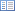Code of Federal Regulations (Last Updated: April 25, 2019)Title 40 - Protection of EnvironmentCHAPTER I — ENVIRONMENTAL PROTECTION AGENCYSUBCHAPTER C — AIR PROGRAMSPart 60 - STANDARDS OF PERFORMANCE FOR NEW STATIONARY SOURCESSubpart LLL — Standards of Performance for Onshore Natural Gas Processing: SO2 Emissions

# § 60.644 Test methods and procedures.

• (a) In conducting the performance tests required in §60.8, the owner or operator shall use as reference methods and procedures the test methods in appendix A of this part or other methods and procedures as specified in this section, except as provided in paragraph §60.8(b).

(b) During a performance test required by §60.8, the owner or operator shall determine the minimum required reduction efficiencies (Z) of SO2 emissions as required in §60.642 (a) and (b) as follows:

(1) The average sulfur feed rate (X) shall be computed as follows:(2) The continuous readings from the process flowmeter shall be used to determine the average volumetric flow rate (Qa) in dscm/day (dscf/day) of the acid gas from the sweetening unit for each run.

(3) The Tutwiler procedure in §60.648 or a chromatographic procedure following ASTM E-260 (incorporated by reference—see §60.17) shall be used to determine the H2S concentration in the acid gas feed from the sweetening unit. At least one sample per hour (at equally spaced intervals) shall be taken during each 4-hour run. The arithmetic mean of all samples shall be the average H2S concentration (Y) on a dry basis for the run. By multiplying the result from the Tutwiler procedure by 1.62 × 10−3, the units gr/100 scf are converted to volume percent.

(4) Using the information from paragraphs (b) (1) and (3), tables 1 and 2 shall be used to determine the required initial (Zi) and continuous (Zc) reduction efficiencies of SO2 emissions.

(c) The owner or operator shall determine compliance with the SO2 standards in §60.642 (a) or (b) as follows:

(1) The emission reduction efficiency (R) achieved by the sulfur recovery technology shall be computed for each run using the following equation:

R = (100 S)/(S + E)

(2) The level indicators or manual soundings shall be used to measure the liquid sulfur accumulation rate in the product storage tanks. Readings taken at the beginning and end of each run, the tank geometry, sulfur density at the storage temperature, and sample duration shall be used to determine the sulfur production rate (S) in kg/hr (lb/hr) for each run.

(3) The emission rate of sulfur shall be computed for each run as follows:(4) The concentration (Ce) of sulfur equivalent shall be the sum of the SO2 and TRS concentrations, after being converted to sulfur equivalents. For each run and each of the test methods specified in this paragraph (c) of this section, the sampling time shall be at least 4 hours. Method 1 shall be used to select the sampling site. The sampling point in the duct shall be at the centroid of the cross-section if the area is less than 5 m2 (54 ft2) or at a point no closer to the walls than 1 m (39 in.) if the cross-sectional area is 5 m2 or more, and the centroid is more than 1 m (39 in.) from the wall.

(i) Method 6 shall be used to determine the SO2 concentration. Eight samples of 20 minutes each shall be taken at 30-minute intervals. The arithmetic average shall be the concentration for the run. The concentration shall be multiplied by 0.5 × 10−3 to convert the results to sulfur equivalent.

(ii) Method 15 shall be used to determine the TRS concentration from reduction-type devices or where the oxygen content of the effluent gas is less than 1.0 percent by volume. The sampling rate shall be at least 3 liters/min (0.1 ft3/min) to insure minimum residence time in the sample line. Sixteen samples shall be taken at 15-minute intervals. The arithmetic average of all the samples shall be the concentration for the run. The concentration in ppm reduced sulfur as sulfur shall be multiplied by 1.333 × 10−3 to convert the results to sulfur equivalent.

(iii) Method 16A or 15 shall be used to determine the reduced sulfur concentration from oxidation-type devices or where the oxygen content of the effluent gas is greater than 1.0 percent by volume. Eight samples of 20 minutes each shall be taken at 30-minute intervals. The arithmetic average shall be the concentration for the run. The concentration in ppm reduced sulfur as sulfur shall be multiplied by 1.333 × 10−3 to convert the results to sulfur equivalent.

(iv) Method 2 shall be used to determine the volumetric flow rate of the effluent gas. A velocity traverse shall be conducted at the beginning and end of each run. The arithmetic average of the two measurements shall be used to calculate the volumetric flow rate (Qsd) for the run. For the determination of the effluent gas molecular weight, a single integrated sample over the 4-hour period may be taken and analyzed or grab samples at 1-hour intervals may be taken, analyzed, and averaged. For the moisture content, two samples of at least 0.10 dscm (3.5 dscf) and 10 minutes shall be taken at the beginning of the 4-hour run and near the end of the time period. The arithmetic average of the two runs shall be the moisture content for the run.

(d) To comply with §60.646(d), the owner or operator shall obtain the information required by using the monitoring devices in paragraph (b) or (c) of this section.

[54 FR 6679, Feb. 14, 1989, as amended at 65 FR 61773, Oct. 17, 2000]

[54 FR 6679, Feb. 14, 1989, as amended at 65 FR 61773, Oct. 17, 2000